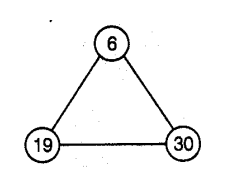# Put three different numbers in the circles,

Question:

Put three different numbers in the circles, so that when you add the numbers at the end of each line, you always get a perfect square.Solution:

$\because \quad 6+19=25$ (perfect square)

$19+30=49$ (perfect square)

and $30+6=36$ (perfect square)